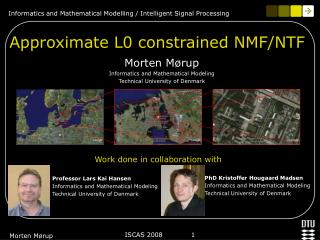DownloadDownload PresentationApproximate L0 constrained NMF/NTF

# Approximate L0 constrained NMF/NTF

Télécharger la présentation## Approximate L0 constrained NMF/NTF

- - - - - - - - - - - - - - - - - - - - - - - - - - - E N D - - - - - - - - - - - - - - - - - - - - - - - - - - -
##### Presentation Transcript

1. Morten Mørup Informatics and Mathematical Modeling Technical University of Denmark Approximate L0 constrained NMF/NTF Work done in collaboration with PhD Kristoffer Hougaard Madsen Informatics and Mathematical Modeling Technical University of Denmark Professor Lars Kai Hansen Informatics and Mathematical Modeling Technical University of Denmark Morten Mørup

2. Non-negative Matrix Factorization (NMF)VWH, V≥0,W≥0, H≥0  (Lee & Seung – Nature 1999) NMF gives Part based representation! Morten Mørup

3. NMF based on Multiplicative updates Step size parameter Morten Mørup

4. fast Non-Negative Least Squares, fNNLS Active Set procedure (Lawson and Hanson, 1974) Morten Mørup

5. NMF not in general unique!! V=WH=(WP)(P-1H)=W’H’ (Donoho & Stodden, 2003) Morten Mørup

6. FIX: Impose sparseness (Hoyer, 2001,2004 Eggert et al. 2004) • Ensures uniqueness • Eases interpretability (sparse representation  factor effects pertain to fewer dimensions) • Can work as model selection(Sparseness can turn off excess factors by letting them become zero) • Resolves over complete representations (when model has many more free variables than data points) L1 used as convex proxy for the L0 norm, i.e. card(H) Morten Mørup

7. Least Angle Regression and Selection(LARS)/Homotopy Method     Morten Mørup

8. Controlling sparsity degree (Patric Hoyer 2004) Controlling sparsity degree (Mørup et al., 2008) Sparsity can now be controlled by evaulating the full regularization path of the NLARS Morten Mørup

9. New Algorithm for sparse NMF: 1: Solve for each column of H using NLARS and obtain solutions for all values of  (i.e. the entire regularization path) 2: Select -solution giving the desired degree of sparsity 3: Update W such that ||Wd||F=1, according to (Eggert et al. 2004) Repeat from step 1 until convergence Morten Mørup

10. CBCL face database USPS handwritten digits Morten Mørup

11. Morten Mørup

12. Conclusion • New efficient algorithm for sparse NMF based on the proposed non-negative version of the LARS algorithm • The obtained full regularization path admit to use L1 as a convex proxy for the L0 norm to control the degree of sparsity given by • The proposed method is more efficient than previous methods to control degree of sparsity. Furhtermore, NLARS is even comparable in speed to the classic efficient fNNLS method. • Proposed method directly generalizes to tensor decompositions through models such as Tucker and PARAFAC when using an alternating least squares approach. Morten Mørup